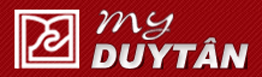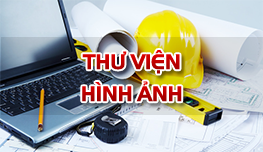Tài liệu học tập

### Bài tập SBVL1 (Tiếng Anh) - Phần TENSION, COMPRESSION and SHEAR (Bài 6 đến bài 10)

• Đăng bởi: lethithanhbinh
• Sinh viên
• 18/10/2018

Tension, compression and shear 6

A bearing pad of the kind used to support machines and bridge girders consists of a linearly elastic material (usually an elastomer, such as rubber) capped by a steel plate (Fig. 6a). Assume that the thickness of the elastomer is h, the dimensions of the plate are a x b, and the pad is subjected to a horizontal shear force V.  Obtain formulas for the average shear stress t(aver) in the elastomer and the horizontal displacement d of the plate (Fig. 6b).

Tension, compression and shear 7

A steel bar serving as a vertical hanger to support heavy machinery in a factory is attached to a support by the bolted  connection shown in Fig. 7. The main part of the hanger has a rectangular cross section with width b1 = 1.5 in. and thickness t = 0.5 in. At the connection the hanger is enlarged to a width b2 = 3.0 in. The bolt, which transfers the load from the hanger to the two gussets, has diameter d = 1.0 in.  Determine the allowable value of the tensile load P in the hanger based upon the following four considerations: (a) The allowable tensile stress in the main part of the hanger is 16,000 psi. (b) The allowable tensile stress in the hanger at its cross section through the bolt hole is 11,000 psi. (The permissible stress at this section is lower because of the stress concentrations around the hole.)  (c) The allowable bearing stress between the hanger and the bolt is 26,000 psi. (d) The allowable shear stress in the bolt is 6,500 psi.

Tension, compression and shear 8

The two-bar truss ABC shown in Fig. 8 has pin supports at points A and C, which are 2.0 m apart. Members AB and BC are steel bars, pin connected at joint B. The length of bar BC is 3.0 m. A sign weighing 5.4 kN is suspended from bar BC at points D and E, which are located 0.8 m and 0.4 m, respectively, from the ends of the bar. Determine the required cross-sectional area of bar AB and the required diameter of the pin at support C if the allowable stresses in tension and shear are 125 MPa and 45 MPa, respectively. (Note: The pins at the supports are in double shear. Also, disregard the weights of members AB and BC.)

Tension, compression and shear 9

A square steel tube of length L= 20 ft and width b2 = 10.0 in. is hoisted by a crane (Fig 9). The tube hangs from a pin of diameter d that is held by the cables at points A and B. The cross section is a hollow square with inner dimension b1= 8.5 in. and outer dimension  b2 =  10.0 in. The allowable shear stress in the pin is 8,700 psi, and the allowable bearing stress between the pin and the tube is 13,000 psi. Determine the minimum diameter of the pin in order to support the weight of the tube. (Note: Disregard the rounded corners of the tube when calculating its weight.)

Tension, compression and shear 10

Two bars of rectangular cross section (thickness t = 15 mm) are connected by a bolt in the manner shown in the Fig 10. The allowable shear stress in the bolt is 90 MPa and the allowable bearing stress between the bolt and the bars is 150 MPa. If the tensile load P _ 31 kN, what is the minimum required diameter dmin of the bolt?

Tải file đính kèm:

Bài viết liên quan